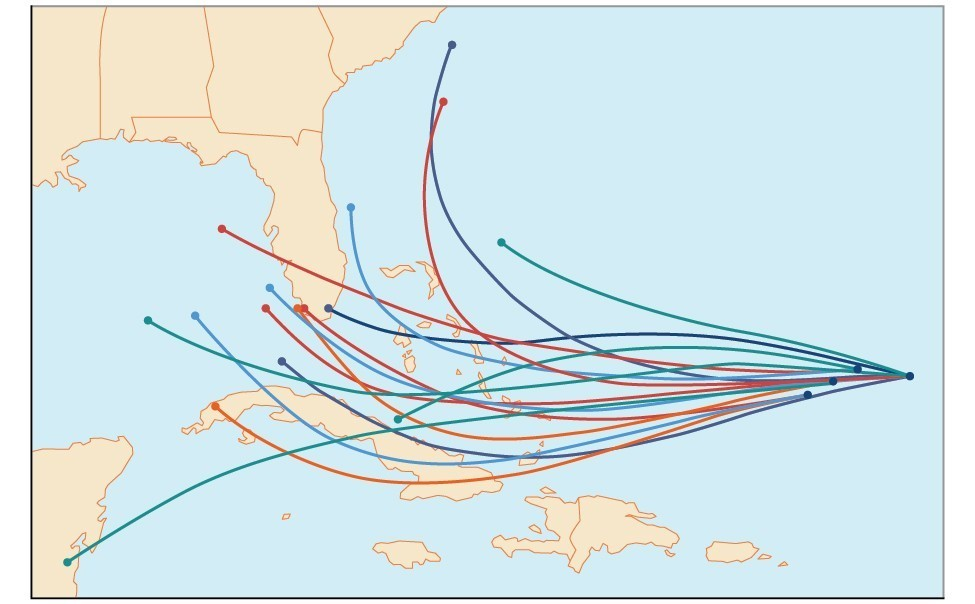## Introduction to Probability

### Learning Objectives

By the end of this section, you will be able to:

• Construct probability models.
• Compute probabilities of equally likely outcomes.
• Compute probabilities of the union of two events.
• Use the complement rule to find probabilities.
• Compute probability using counting theory.Figure 1. An example of a “spaghetti model,” which can be used to predict possible paths of a tropical storm.

Residents of the Southeastern United States are all too familiar with charts, known as spaghetti models, such as the one in Figure 1. They combine a collection of weather data to predict the most likely path of a hurricane. Each colored line represents one possible path. The group of squiggly lines can begin to resemble strands of spaghetti, hence the name. In this section, we will investigate methods for making these types of predictions.

1. The figure is for illustrative purposes only and does not model any particular storm.